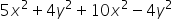Mathematics
Easy

Question

### 15x2    16x2    8y2    4y2Hint:

## The correct answer is: 15x2

### The given expression is 5x2+ 4y2+ 10x2– 4y2There are two variables in the above expression. The variables are: ‘x’ and ‘y’. There are four terms in the above expression. We have to perform the operations between them and find the right answer.If we see, the first and third terms contain x raised to power two. And the second and fourth terms contains y raised to power 2. Such terms with the same variables or same variables raised to same power are called “like terms.” And the terms with different variables are called “unlike terms.”For like terms, we can perform the operations between their coefficients by keeping the variable constant. It means we can treat each term as a constant and perform operations like normal operations on constants.For the above expression, we can perform an operation between the first and third terms as they have the same variable ‘x^2’. And the second and fourth term as they have the same variable ‘y^2’, so we can perform the operation directly.Let’s rearrange the terms to bring the like terms together.5x2+ 4y2 + 10x2– 4y2 =( 5+10)x2 + (4-4)y2For first bracket, we will keep x^2 constant and add 5 and 10. For second bracket we will keep y^ 2 constant. And subtract 4 from 4.5x2 + 4y2+ 10x2– 4y2 =(15)x2 + (0)y2=15x2       Therefore, the value of the equation is 15x2So option which is ’15x2', is the right option.

We have to be careful about the powers of the variables too. The terms with same variable having same power are only operated with each other.

### Related Questions to study#### With Turito Foundation.#### Get an Expert Advice From Turito.